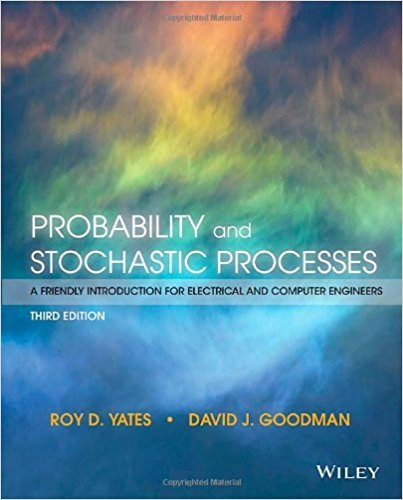×
×

# Solutions for Chapter 9: Sums of Random Variables## Full solutions for Probability and Stochastic Processes: A Friendly Introduction for Electrical and Computer Engineers | 3rd Edition

ISBN: 9781118324561Solutions for Chapter 9: Sums of Random Variables

Solutions for Chapter 9
4 5 0 313 Reviews
18
0
##### ISBN: 9781118324561

This expansive textbook survival guide covers the following chapters and their solutions. Since 49 problems in chapter 9: Sums of Random Variables have been answered, more than 11174 students have viewed full step-by-step solutions from this chapter. Probability and Stochastic Processes: A Friendly Introduction for Electrical and Computer Engineers was written by and is associated to the ISBN: 9781118324561. This textbook survival guide was created for the textbook: Probability and Stochastic Processes: A Friendly Introduction for Electrical and Computer Engineers, edition: 3. Chapter 9: Sums of Random Variables includes 49 full step-by-step solutions.

Key Statistics Terms and definitions covered in this textbook
• 2 k p - factorial experiment

A fractional factorial experiment with k factors tested in a 2 ? p fraction with all factors tested at only two levels (settings) each

• Assignable cause

The portion of the variability in a set of observations that can be traced to speciic causes, such as operators, materials, or equipment. Also called a special cause.

• Asymptotic relative eficiency (ARE)

Used to compare hypothesis tests. The ARE of one test relative to another is the limiting ratio of the sample sizes necessary to obtain identical error probabilities for the two procedures.

• Attribute control chart

Any control chart for a discrete random variable. See Variables control chart.

• Average run length, or ARL

The average number of samples taken in a process monitoring or inspection scheme until the scheme signals that the process is operating at a level different from the level in which it began.

• Bivariate normal distribution

The joint distribution of two normal random variables

• Block

In experimental design, a group of experimental units or material that is relatively homogeneous. The purpose of dividing experimental units into blocks is to produce an experimental design wherein variability within blocks is smaller than variability between blocks. This allows the factors of interest to be compared in an environment that has less variability than in an unblocked experiment.

• Central composite design (CCD)

A second-order response surface design in k variables consisting of a two-level factorial, 2k axial runs, and one or more center points. The two-level factorial portion of a CCD can be a fractional factorial design when k is large. The CCD is the most widely used design for itting a second-order model.

• Chi-square (or chi-squared) random variable

A continuous random variable that results from the sum of squares of independent standard normal random variables. It is a special case of a gamma random variable.

• Chi-square test

Any test of signiicance based on the chi-square distribution. The most common chi-square tests are (1) testing hypotheses about the variance or standard deviation of a normal distribution and (2) testing goodness of it of a theoretical distribution to sample data

• Conditional probability density function

The probability density function of the conditional probability distribution of a continuous random variable.

• Conditional probability mass function

The probability mass function of the conditional probability distribution of a discrete random variable.

• Consistent estimator

An estimator that converges in probability to the true value of the estimated parameter as the sample size increases.

• Continuous random variable.

A random variable with an interval (either inite or ininite) of real numbers for its range.

• Crossed factors

Another name for factors that are arranged in a factorial experiment.

• Discrete uniform random variable

A discrete random variable with a inite range and constant probability mass function.

• Event

A subset of a sample space.

• F-test

Any test of signiicance involving the F distribution. The most common F-tests are (1) testing hypotheses about the variances or standard deviations of two independent normal distributions, (2) testing hypotheses about treatment means or variance components in the analysis of variance, and (3) testing signiicance of regression or tests on subsets of parameters in a regression model.

• Fraction defective control chart

See P chart

• Hat matrix.

In multiple regression, the matrix H XXX X = ( ) ? ? -1 . This a projection matrix that maps the vector of observed response values into a vector of itted values by yˆ = = X X X X y Hy ( ) ? ? ?1 .

×